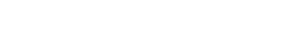# Probabilistic Modeling and Uncertainty Quantification

Quantifying the level of uncertainty is an important facet of modeling complex heterogeneous systems. Uncertainty quantification (UQ) is the process of identifying accurate estimates of probabilistic measures for a given model, with a finite, computationally feasible number of model evaluations. In most cases, uncertainty quantification approaches are constructed on the basis of multiple simulations, in which parameters are obtained by sampling from the probability distributions describing all physical variables. This can be achieved in a number of ways, for example:

• Reducing the number of random variables required to represent the inherent uncertainties in the system: for example, Polynomial Chaos expansions provide a means for reducing an infinite number of correlated random variables describing a system to an optimally selected finite number of random variables .
• Minimize the number of required model evaluations by maximizing the information obtained from each and every model evaluation and/or experiment:  for example, McDiarmid’s inequality provides a bound on probability of failure that is established through optimization approaches that identify the design point furthest from the expected response [91,92].
• Probing model parameters that have greatest impact on system uncertainty:  for example, Bayesian methods provide a means for updating probability distributions given new information and for identifying parameters that most affect system response uncertainty.

In the proposed efforts, model evaluation via stochastic simulation (a process by which the parameter vector sampled from the random dimension are generated and applied to the model, all in the context of an uncertainty quantification framework) is the underlying tool for uncertainty quantification.. Materials studied in CEIMM exhibit uncertainties at every length scale. These random variations in material structure are manifested in stochastic localizations that initiate and/or propagate failure. Therefore, stochastic simulation in the context of failure models must be based on mechanically rigorous parameterization of the heterogeneous structure at multiple scales. The parameterization process yields a very large number of random variables, some exhibiting strong correlations and/or very high scatter, significantly complicating stochastic simulation. A significant aspect of CEIMM research is to apply stochastic mechanics to incorporate the data from advanced imaging techniques into the advanced modeling capabilities.#### Johns Hopkins University, Whiting School of Engineering

3400 North Charles Street, Baltimore, MD 21218-2608

• 410-516-5033